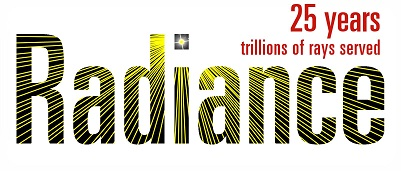Hi all,
I have a .ies file of a lamp with a color temperature of ca. 1850K.
I found this topic https://discourse.radiance-online.org/t/ies2rad-question-specifying-color-temperature/3568 but I am not sure how to proceed to assign the color.

Greg was suggesting:

ies2rad -df -m 0.88 -t default -c my_red my_green my_blue

The red, green and blue values will be multiplied by the 0.88 factor, so I guess you want the RGB to normalize to 1.0. To get these from CIE (x,y) chromaticities, you can use rcalc like so:

rcalc -n -e “ix=…;iy=…;” -f xyz_rgb.cal -e “Xi=ix/iy;Yi=1;Zi=(1-ix-iy)/iy” -e “\$1=R(Xi,Yi,Zi);\$2=G(Xi,Yi,Zi);\$3=B(Xi,Yi,Zi)”

The file “xyz_rgb.cal” is in the standard distribution in src/cal/cal/, along with other files like it. Put your desired (x,y) coordinates in the first setting, e.g., -e “ix=0.3153;iy=0.3417”. (On Unix, replace the double-quotes with single-quotes above.)

This is the procedure I would like to follow:

I first turn “1850K” into to CIE 1931 xy using a calculator (is there any reliable one you can suggest?)

Then I run rcalc to turn CIE values into RGB ones. I then past and copy those values as “my_red my_green my_blue” and execute ies2rad.

1. Would this be correct?

2. Do we use .88 to rescale the RBG values to 0-1?

3. I see that Greg suggest using :

rcalc -f blackbody.cal -e T=2700 -e “\$1=cct_x(T);\$2=cct_y(T)”

for K to CXY translation. In my case, the lamp is a simple flame. Would you recommend it?